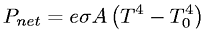Equations > Physics > Thermodynamics > Net power radiated by an object

### Net power radiated by an objectLatex Code:

MathML Code:

 ${P}_{\mathrm{net}}=e\sigma A\left({T}^{4}-{T}_{0}^{4}\right)$

MathType 5.0: